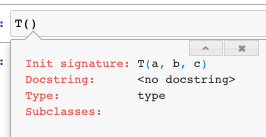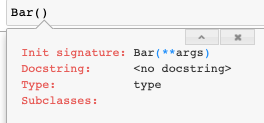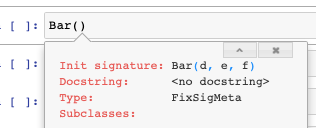# Meta

Metaclasses
``````from fastcore.foundation import *
from nbdev.showdoc import *
from fastcore.nb_imports import *``````

source

### test_sig

`` test_sig (f, b)``

Test the signature of an object

``````def func_1(h,i,j): pass
def func_2(h,i=3, j=[5,6]): pass

class T:
def __init__(self, a, b): pass

test_sig(func_1, '(h, i, j)')
test_sig(func_2, '(h, i=3, j=[5, 6])')
test_sig(T, '(a, b)')``````

source

### FixSigMeta

`` FixSigMeta (name, bases, dict)``

A metaclass that fixes the signature on classes that override `__new__`

When you inherit from a class that defines `__new__`, or a metaclass that defines `__call__`, the signature of your `__init__` method is obfuscated such that tab completion no longer works. `FixSigMeta` fixes this issue and restores signatures.

To understand what `FixSigMeta` does, it is useful to inspect an object’s signature. You can inspect the signature of an object with `inspect.signature`:

``````class T:
def __init__(self, a, b, c): pass

inspect.signature(T)``````
``<Signature (a, b, c)>``

This corresponds to tab completion working in the normal way:However, when you inherhit from a class that defines `__new__` or a metaclass that defines `__call__` this obfuscates the signature by overriding your class with the signature of `__new__`, which prevents tab completion from displaying useful information:

``````class Foo:
def __new__(self, **args): pass

class Bar(Foo):
def __init__(self, d, e, f): pass

inspect.signature(Bar)``````
``<Signature (d, e, f)>``Finally, the signature and tab completion can be restored by inheriting from the metaclass `FixSigMeta` as shown below:

``````class Bar(Foo, metaclass=FixSigMeta):
def __init__(self, d, e, f): pass

test_sig(Bar, '(d, e, f)')
inspect.signature(Bar)``````
``<Signature (d, e, f)>``If you need to define a metaclass that overrides `__call__` (as done in `PrePostInitMeta`), you need to inherit from `FixSigMeta` instead of `type` when constructing the metaclass to preserve the signature in `__init__`. Be careful not to override `__new__` when doing this:

``````class TestMeta(FixSigMeta):
# __new__ comes from FixSigMeta
def __call__(cls, *args, **kwargs): pass

class T(metaclass=TestMeta):
def __init__(self, a, b): pass

test_sig(T, '(a, b)')``````

On the other hand, if you fail to inherit from `FixSigMeta` when inheriting from a metaclass that overrides `__call__`, your signature will reflect that of `__call__` instead (which is often undesirable):

``````class GenericMeta(type):
"A boilerplate metaclass that doesn't do anything for testing."
def __new__(cls, name, bases, dict):
return super().__new__(cls, name, bases, dict)
def __call__(cls, *args, **kwargs): pass

class T2(metaclass=GenericMeta):
def __init__(self, a, b): pass

# We can avoid this by inheriting from the metaclass `FixSigMeta`
test_sig(T2, '(*args, **kwargs)')``````

source

### PrePostInitMeta

`` PrePostInitMeta (name, bases, dict)``

A metaclass that calls optional `__pre_init__` and `__post_init__` methods

`__pre_init__` and `__post_init__` are useful for initializing variables or performing tasks prior to or after `__init__` being called, respectively. Fore example:

``````class _T(metaclass=PrePostInitMeta):
def __pre_init__(self):  self.a  = 0;
def __init__(self,b=0):  self.b = self.a + 1; assert self.b==1
def __post_init__(self): self.c = self.b + 2; assert self.c==3

t = _T()
test_eq(t.a, 0) # set with __pre_init__
test_eq(t.b, 1) # set with __init__
test_eq(t.c, 3) # set with __post_init__``````

One use for `PrePostInitMeta` is avoiding the `__super__().__init__()` boilerplate associated with subclassing, such as used in `AutoInit`.

source

### AutoInit

`` AutoInit (*args, **kwargs)``

Same as `object`, but no need for subclasses to call `super().__init__`

This is normally used as a mixin, eg:

``````class TestParent():
def __init__(self): self.h = 10

class TestChild(AutoInit, TestParent):
def __init__(self): self.k = self.h + 2

t = TestChild()
test_eq(t.h, 10) # h=10 is initialized in the parent class
test_eq(t.k, 12)``````

source

### NewChkMeta

`` NewChkMeta (name, bases, dict)``

Metaclass to avoid recreating object passed to constructor

`NewChkMeta` is used when an object of the same type is the first argument to your class’s constructor (i.e. the `__init__` function), and you would rather it not create a new object but point to the same exact object.

This is used in `L`, for example, to avoid creating a new object when the object is already of type `L`. This allows the users to defenisvely instantiate an `L` object and just return a reference to the same object if it already happens to be of type `L`.

For example, the below class `_T` optionally accepts an object `o` as its first argument. A new object is returned upon instantiation per usual:

``````class _T():
"Testing"
def __init__(self, o):
# if `o` is not an object without an attribute `foo`, set foo = 1
self.foo = getattr(o,'foo',1)``````
``````t = _T(3)
test_eq(t.foo,1) # 1 was not of type _T, so foo = 1

t2 = _T(t) #t1 is of type _T
assert t is not t2 # t1 and t2 are different objects``````

However, if we want `_T` to return a reference to the same object when passed an an object of type `_T` we can inherit from the `NewChkMeta` class as illustrated below:

``````class _T(metaclass=NewChkMeta):
"Testing with metaclass NewChkMeta"
def __init__(self, o=None, b=1):
# if `o` is not an object without an attribute `foo`, set foo = 1
self.foo = getattr(o,'foo',1)
self.b = b``````

We can now test `t` and `t2` are now pointing at the same object when using this new definition of `_T`:

``````t = _T(3)
test_eq(t.foo,1) # 1 was not of type _T, so foo = 1

t2 = _T(t) # t2 will now reference t

test_is(t, t2) # t and t2 are the same object
t2.foo = 5 # this will also change t.foo to 5 because it is the same object
test_eq(t.foo, 5)
test_eq(t2.foo, 5)``````

However, there is one exception to how `NewChkMeta` works. If you pass any additional arguments in the constructor a new object is returned, even if the first object is of the same type. For example, consider the below example where we pass the additional argument `b` into the constructor:

``````t3 = _T(t, b=1)
assert t3 is not t

t4 = _T(t) # without any arguments the constructor will return a reference to the same object
assert t4 is t``````

Finally, it should be noted that `NewChkMeta` as well as all other metaclases in this section, inherit from `FixSigMeta`. This means class signatures will always be preserved when inheriting from this metaclass (see docs for `FixSigMeta` for more details):

``test_sig(_T, '(o=None, b=1)')``

source

### BypassNewMeta

`` BypassNewMeta (name, bases, dict)``

Metaclass: casts `x` to this class if it’s of type `cls._bypass_type`

`BypassNewMeta` is identical to `NewChkMeta`, except for checking for a class as the same type, we instead check for a class of type specified in attribute `_bypass_type`.

In NewChkMeta, objects of the same type passed to the constructor (without arguments) would result into a new variable referencing the same object. However, with `BypassNewMeta` this only occurs if the type matches the `_bypass_type` of the class you are defining:

``````class _TestA: pass
class _TestB: pass

class _T(_TestA, metaclass=BypassNewMeta):
_bypass_type=_TestB
def __init__(self,x): self.x=x``````

In the below example, `t` does not refer to `t2` because `t` is of type `_TestA` while `_T._bypass_type` is of type `TestB`:

``````t = _TestA()
t2 = _T(t)
assert t is not t2``````

However, if `t` is set to `_TestB` to match `_T._bypass_type`, then both `t` and `t2` will refer to the same object.

``````t = _TestB()
t2 = _T(t)
t2.new_attr = 15

test_is(t, t2)
# since t2 just references t these will be the same
test_eq(t.new_attr, t2.new_attr)

# likewise, chaning an attribute on t will also affect t2 because they both point to the same object.
t.new_attr = 9
test_eq(t2.new_attr, 9)``````

## Metaprogramming

source

### empty2none

`` empty2none (p)``

Replace `Parameter.empty` with `None`

source

### anno_dict

`` anno_dict (f)``

`__annotation__ dictionary with`empty`cast to`None`, returning empty if doesn’t exist

``````def _f(a:int, b:L)->str: ...
test_eq(anno_dict(_f), {'a': int, 'b': L, 'return': str})``````

source

### use_kwargs_dict

`` use_kwargs_dict (keep=False, **kwargs)``

Decorator: replace `**kwargs` in signature with `names` params

Replace all `**kwargs` with named arguments like so:

``````@use_kwargs_dict(y=1,z=None)
def foo(a, b=1, **kwargs): pass

test_sig(foo, '(a, b=1, *, y=1, z=None)')``````

Add named arguments, but optionally keep `**kwargs` by setting `keep=True`:

``````@use_kwargs_dict(y=1,z=None, keep=True)
def foo(a, b=1, **kwargs): pass

test_sig(foo, '(a, b=1, *, y=1, z=None, **kwargs)')``````

source

### use_kwargs

`` use_kwargs (names, keep=False)``

Decorator: replace `**kwargs` in signature with `names` params

`use_kwargs` is different than `use_kwargs_dict` as it only replaces `**kwargs` with named parameters without any default values:

``````@use_kwargs(['y', 'z'])
def foo(a, b=1, **kwargs): pass

test_sig(foo, '(a, b=1, *, y=None, z=None)')``````

You may optionally keep the `**kwargs` argument in your signature by setting `keep=True`:

``````@use_kwargs(['y', 'z'], keep=True)
def foo(a, *args, b=1, **kwargs): pass
test_sig(foo, '(a, *args, b=1, y=None, z=None, **kwargs)')``````

source

### delegates

`` delegates (to:function=None, keep=False, but:list=None)``

Decorator: replace `**kwargs` in signature with params from `to`

Type Default Details
to function None Delegatee
keep bool False Keep `kwargs` in decorated function?
but list None Exclude these parameters from signature

A common Python idiom is to accept `**kwargs` in addition to named parameters that are passed onto other function calls. It is especially common to use `**kwargs` when you want to give the user an option to override default parameters of any functions or methods being called by the parent function.

For example, suppose we have have a function `foo` that passes arguments to `baz` like so:

``````def baz(a, b:int=2, c:int=3): return a + b + c

def foo(c, a, **kwargs):
return c + baz(a, **kwargs)

assert foo(c=1, a=1) == 7``````

The problem with this approach is the api for `foo` is obfuscated. Users cannot introspect what the valid arguments for `**kwargs` are without reading the source code. When a user tries tries to introspect the signature of `foo`, they are presented with this:

``inspect.signature(foo)``
``<Signature (c, a, **kwargs)>``

We can address this issue by using the decorator `delegates` to include parameters from other functions. For example, if we apply the `delegates` decorator to `foo` to include parameters from `baz`:

``````@delegates(baz)
def foo(c, a, **kwargs):
return c + baz(a, **kwargs)

test_sig(foo, '(c, a, *, b: int = 2)')
inspect.signature(foo)``````
``<Signature (c, a, *, b: int = 2)>``

We can optionally decide to keep `**kwargs` by setting `keep=True`:

``````@delegates(baz, keep=True)
def foo(c, a, **kwargs):
return c + baz(a, **kwargs)

inspect.signature(foo)``````
``<Signature (c, a, *, b: int = 2, **kwargs)>``

It is important to note that only parameters with default parameters are included. For example, in the below scenario only `c`, but NOT `e` and `d` are included in the signature of `foo` after applying `delegates`:

``````def basefoo(e, d, c=2): pass

@delegates(basefoo)
def foo(a, b=1, **kwargs): pass
inspect.signature(foo) # e and d are not included b/c they don't have default parameters.``````
``<Signature (a, b=1, *, c=2)>``

The reason that required arguments (i.e. those without default parameters) are automatically excluded is that you should be explicitly implementing required arguments into your function’s signature rather than relying on `delegates`.

Additionally, you can exclude specific parameters from being included in the signature with the `but` parameter. In the example below, we exclude the parameter `d`:

``````def basefoo(e, c=2, d=3): pass

@delegates(basefoo, but= ['d'])
def foo(a, b=1, **kwargs): pass

test_sig(foo, '(a, b=1, *, c=2)')
inspect.signature(foo)``````
``<Signature (a, b=1, *, c=2)>``

You can also use `delegates` between methods in a class. Here is an example of `delegates` with class methods:

``````# example 1: class methods
class _T():
@classmethod
def foo(cls, a=1, b=2):
pass

@classmethod
@delegates(foo)
def bar(cls, c=3, **kwargs):
pass

test_sig(_T.bar, '(c=3, *, a=1, b=2)')``````

Here is the same example with instance methods:

``````# example 2: instance methods
class _T():
def foo(self, a=1, b=2):
pass

@delegates(foo)
def bar(self, c=3, **kwargs):
pass

t = _T()
test_sig(t.bar, '(c=3, *, a=1, b=2)')``````

You can also delegate between classes. By default, the `delegates` decorator will delegate to the superclass:

``````class BaseFoo:
def __init__(self, e, c=2): pass

@delegates()# since no argument was passsed here we delegate to the superclass
class Foo(BaseFoo):
def __init__(self, a, b=1, **kwargs): super().__init__(**kwargs)

test_sig(Foo, '(a, b=1, *, c=2)')``````

source

### method

`` method (f)``

Mark `f` as a method

The `method` function is used to change a function’s type to a method. In the below example we change the type of `a` from a function to a method:

``````def a(x=2): return x + 1
assert type(a).__name__ == 'function'

a = method(a)
assert type(a).__name__ == 'method'``````

source

### funcs_kwargs

`` funcs_kwargs (as_method=False)``

Replace methods in `cls._methods` with those from `kwargs`

The `func_kwargs` decorator allows you to add a list of functions or methods to an existing class. You must set this list as a class attribute named `_methods` when defining your class. Additionally, you must incldue the `**kwargs` argument in the `___init__` method of your class.

After defining your class this way, you can add functions to your class upon instantation as illusrated below.

For example, we define class `T` to allow adding the function `b` to class `T` as follows (note that this function is stored as an attribute of `T` and doesn’t have access to `cls` or `self`):

``````@funcs_kwargs
class T:
_methods=['b'] # allows you to add method b upon instantiation
def __init__(self, f=1, **kwargs): pass # don't forget to include **kwargs in __init__
def a(self): return 1
def b(self): return 2

t = T()
test_eq(t.a(), 1)
test_eq(t.b(), 2)``````

Because we defined the class `T` this way, the signature of `T` indicates the option to add the function or method(s) specified in `_methods`. In this example, `b` is added to the signature:

``````test_sig(T, '(f=1, *, b=None)')
inspect.signature(T)``````
``<Signature (f=1, *, b=None)>``

You can now add the function `b` to class `T` upon instantiation:

``````def _new_func(): return 5

t = T(b = _new_func)
test_eq(t.b(), 5)``````

If you try to add a function with a name not listed in `_methods` it will be ignored. In the below example, the attempt to add a function named `a` is ignored:

``````t = T(a = lambda:3)
test_eq(t.a(), 1) # the attempt to add a is ignored and uses the original method instead.``````

Note that you can also add methods not defined in the original class as long it is specified in the `_methods` attribute:

``````@funcs_kwargs
class T:
_methods=['c']
def __init__(self, f=1, **kwargs): pass

t = T(c = lambda: 4)
test_eq(t.c(), 4)``````

Until now, these examples showed how to add functions stored as an instance attribute without access to `self`. However, if you need access to `self` you can set `as_method=True` in the `func_kwargs` decorator to add a method instead:

``````def _f(self,a=1): return self.num + a # access the num attribute from the instance

@funcs_kwargs(as_method=True)
class T:
_methods=['b']
num = 5

t = T(b = _f) # adds method b
test_eq(t.b(5), 10) # self.num + 5 = 10``````

Here is an example of how you might use this functionality with inheritence:

``````def _f(self,a=1): return self.num * a #multiply instead of add

class T2(T):
def __init__(self,num):
super().__init__(b = _f) # add method b from the super class
self.num=num

t = T2(num=3)
test_eq(t.b(a=5), 15) # 3 * 5 = 15
test_sig(T2, '(num)')``````